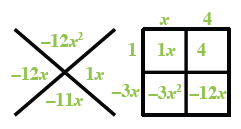### Home > INT3 > Chapter 2 > Lesson 2.2.5 > Problem2-130

2-130.

For the function $h(x)=-3x^2-11x+4$, determine the following values.

1. $h(0)$

Substitute $0$ for $x$ and simplify.

$h(0)=−3(0)^2−11(0)+4$

$h(0)=4$

1. $h(2)$

See part (a).

$h(2)=−30$

1. $h(-1)$

Be careful of the signs when multiplying negative numbers.

$h(−1)=12$

1. $h\left(\frac{1}{2}\right)$

Remember how to multiply fractions.

1. For what value(s) of $x$ does $h(x)=0$?

$0=−3x^2−11x+4$